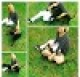# Place Value Part 4 — Multiplication

In this post, I will focus on some strategies for multiplying double digit numbers (whole and decimal):  using manipulatives, the area model, partial products, and the bowtie method.  I highly recommend helping students learn these methods BEFORE the standard algorithm because it is highly linked to number sense and place value. With these methods, students should see the magnitude of the number and increase their understanding of estimation as well as the ability to determine the reasonableness of their answer. Then, when they are very versed with these methods, learn the standard algorithm and compare side by side to see how they all have the same information, but in different format. Students then have a choice of how to solve (or to use two strategies to check their answers). Try my “Choose 3 Ways” work mat as bell work or ticket in the door. Get it free here.

Using Manipulatives:  Using base ten pieces is helpful to see that multiplication means equal groups. Students build understanding at the concrete and pictorial levels first. The following free virutal manipulatives are from didax. The good thing about these is you don’t have to worry about having enough pieces for everyone!!:  https://www.didax.com/math/virtual-manipulatives.htmlArea Model: This method can be illustrated with base ten manipulatives for a concrete experience. Using a frame for a multiplication table, show the two factors on each corner (see examples below for 60 x 5 and 12 x 13). Then fill in the inside of the frame with base ten pieces that match the size of the factors. You must end up making a complete square or rectangle. This makes it relatively easy to see and count the parts: 60 x 3 and 5 x 3 for the first problem and (10 x 10) + (3 x 10) + (2 x 10) + (2 x 3) for the second. I’ve included a larger problem (65 x 34) in case you are curious what that looks like. The first 2 could be managed by students with materials you have in class, but I doubt you want to tackle the last one with individual students – nor do you probably have that many base ten pieces. A drawing or model would be preferred in that case. The point of the visual example is then to connect to the boxed method of the area model, which I have shown in blank form in the examples . . . and with pictures below. I also included a photo from another good strategy I saw on google images (sorry, I don’t know the author) which also shows 12 x 13 using graph paper.

For the boxed area model, break up the factors by place value. If multiplying with a one-digit number, you only need 1 row. If multiplying 2 digits by 2 digits, you need 2 rows.  3 digit by 2 digit would need 3 columns and 2 rows. Then multiply each part together and add the partial products. For double digits, the adding can be done vertically or horizontally (using mental math).

With this model, students get to practice multiplying by groups of 10.  As shown:  60 x 3 . . . think of 6 x 3 = 18, then multiply by 10 (add a zero) to get 180. For 60 x 30, think of 6 x 3 = 18, then multiply by 100 (add 2 zeroes) to get 1800.

Partial Products: This model produces the same partial products as the area model, but in a more algorithmic looking format (vertically). Students take apart the factors, but instead of combining multiplication with regrouping / adding within the problem, all of the products are listed below, lined up in columns of course. Again, this method helps students think of what each part of the multiplication problem means, with heavy emphasis on place value and realizing that in the problem 65 x 3, it’s not 6 x 3 but 60 x 3. For students, using lined notebook paper turned sideways helps keep columns lined up – or graph paper helps too. Examples:

Bowtie / F.O.I.L. Method: In case you have seen this model ciruculated on Pinterest, you will see the same information as the area model and partial products, but the visual of the lines on the bowtie might help make sure all factors are multiplied. Break apart the factors by place value and put one across the top and the other across the bottom. Then start at one corner, follow the lines, and multiply the two numbers at each end of the line together. Stop when you get back to where you started. Add all of the products together.The F.O.I.L. method is a term students will hear more in algebra regarding multiplying binomials, but it applies when multiplying two double-digit numbers together also. F = First (multiply the first two numbers in each set of numbers); O = Outside (multiply the two outside numbers); I = Inside (multiply the two inside numbers); and L = Last (multiply the last two digits, which are the ones).

Have a great week!! Next post will be place value regarding division.

Interested in tutoring individual or small groups of students? You may be interested in Varsity Tutors. You can choose your hours and the students you would like to work with. See the link with my picture. If you are interested, use this link and each of us gets a little bonus!

## One thought on “Place Value Part 4 — Multiplication”

1.Tricia

Great website. Interested in Varsity Tutoring.